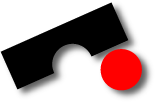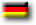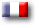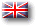Dies ist ein Archiv des alten Softwaretechnik Lehrstuhls der Universität des Saarlandes. Es ist nicht länger aktuell.# Software MiningSS2007

Lehrstuhl für Softwaretechnik (Prof. Zeller)
Universität des Saarlandes – Informatik
Informatik Campus des Saarlandes
Campus E9 1 (CISPA)
66123 Saarbrücken
E-mail: zeller @ cs.uni-saarland.de
Telefon: +49 681 302-70970### Exercise 1 - Introductory Data Analysis (8th May '07)

• Calculate the mean, median and mode of Project Effort and Length [Verzani, Section 2].
• Calculate the Pearsons and Spearmans correlation between Project Effort and Length. Can you explain the difference [Verzani, pp. 26]?
• Build a linear regression model using Length as the independent variable and Project Effort as dependent [Verzani, pp. 28].
• Search the conditions that the data must meet to be used in a linear regression model. Summarise your findings in a bulleted list. Can you check if the conditions hold true for the regression model built in the previous step?
Summarise the above your results (in groups of two) and submit them, preferably as PDFs, to Rahul Premraj on 7th May latest by 4PM.

### Exercise 2 - Linear Regression (postponed until 22th May '07)

We more or less repeat last week's exercises, but with some extenions/modifications. Your list of tasks to accomplish this week are as follows:
• Rebuild the linear regression model using Effort as the dependent variable and Length as indepdent. However, this time, convert both features into their natural logarithms (not log to the base 10) first. Compare the R-square values of both models. Comment on which model do you think is better.
• Divide your data into a training and testing (2:1 ratio) set by randomly allocating projects to the two groups. Remember to filter out the 4 incomplete projects first. Do this only once, in contrast to what we discussed in the Exercises class.
• Rebuild linear regression models using Effort as the dependent variable and Length as indepdent (with and without log transformations) using the training set. Using the predict function in R, predict the Effort value of projects in the test set. Compare the prediction accuracy of each model. Remember that for the log model, you will have to re-transform the prediction value of Effort into its anti-log form. You can do this by issuing the command e^(log-value) where e is a constant and its value is 2.718.
• Build a linear regression model on the training data using Effort as the dependent variable and all other variables (except Project ID) as independent. Take only the raw form of the data, i.e. no log transformations. Comment on the prediction accuracy of this model and compare it to the ones above. Any thoughts on the effect of having Language as an independent variable in this model?
• In your exercise sheets, include the indices of the projects that comprise your training and testing set in the appendix.

### Exercise 3 - Dummy Variables and Stepwise Regression (due on 5th June by 8am)

You are required to undertake the following tasks this week -
• Build a linear regression model by substituting the Language feature by dummy variables. Use the same training and testing data from Exercise 2.
• Build a backward elimination stepwise regression model, again using dummy variables for the Language feature.
• Compare the prediction accuracy of both models by predicting projects in your testing set using sum of residuals as an indicator.

<premraj@cs.uni-saarland.de> · http://www.st.cs.uni-saarland.de/edu/softmine2007/exercises.php · Stand: 2018-04-05 13:40